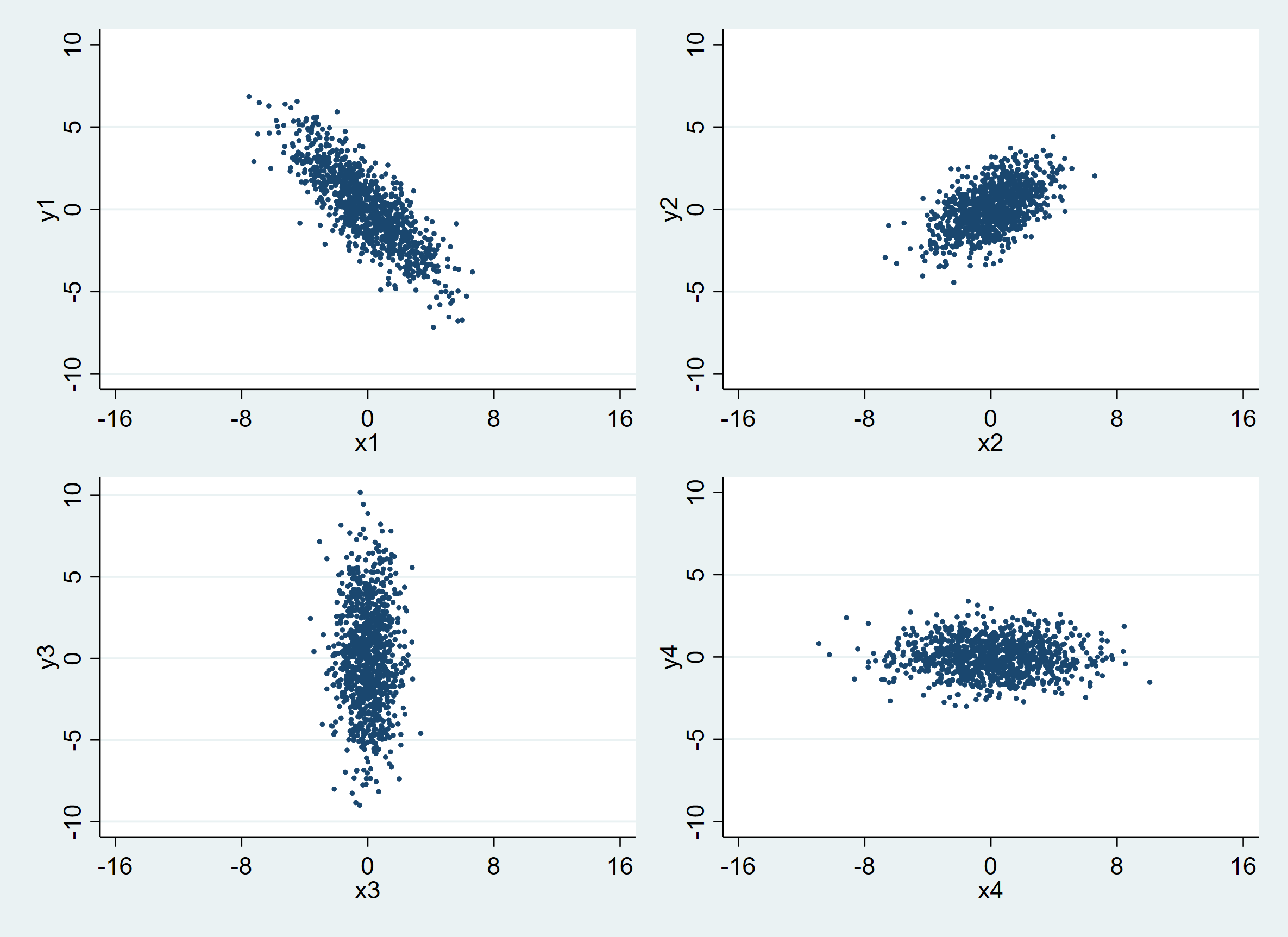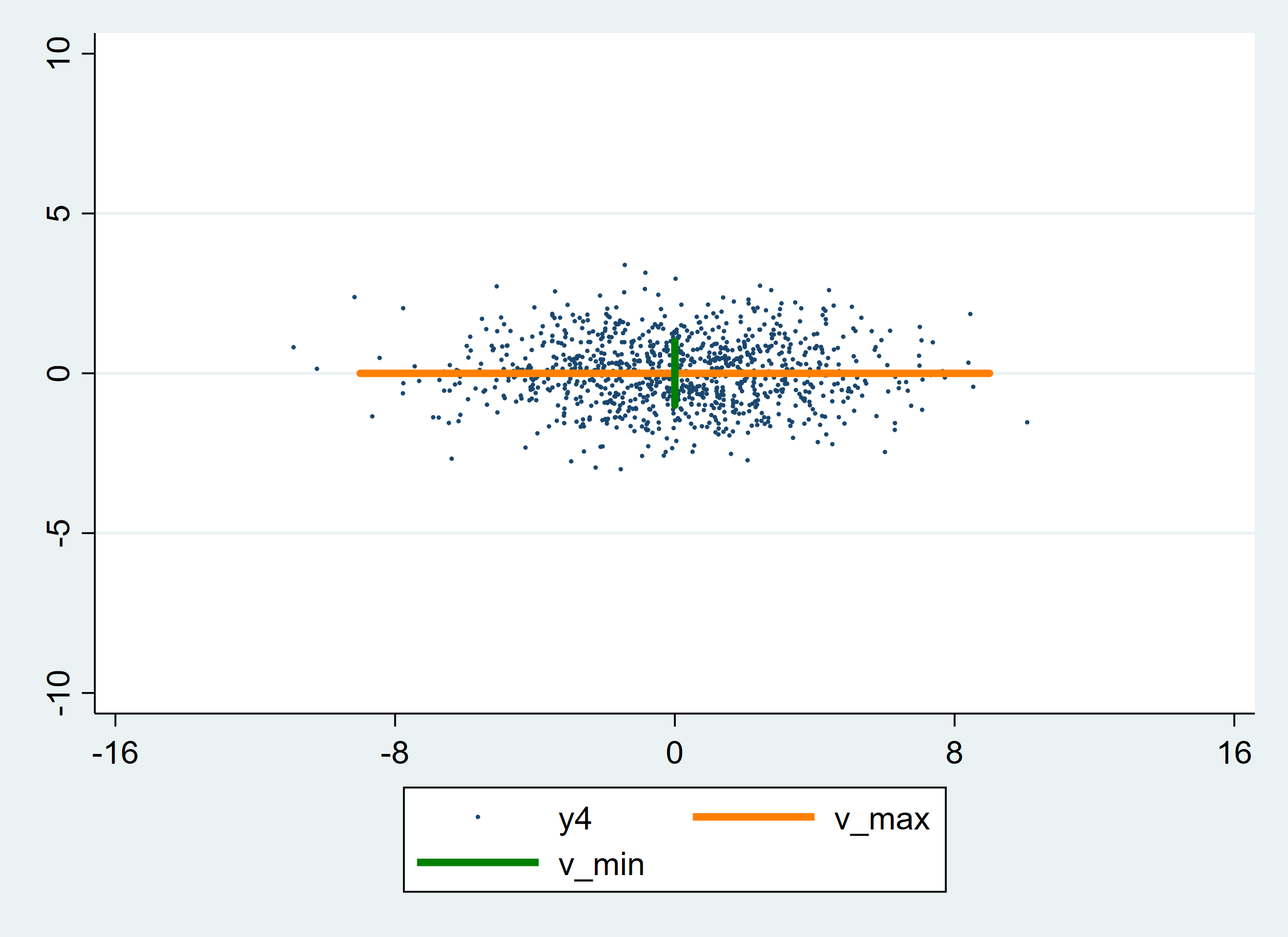# 主成分分析-交互固定效应基础：协方差矩阵的几何意义

Stata连享会   主页 || 视频 || 推文 || 知乎 || Bilibili 站

New！ lianxh 命令发布了：

. ssc install lianxh

. help lianxh

⛳ Stata 系列推文：

## 1. 引言

### 1.2 协方差矩阵的特征值分解有什么用？

1. 计算原数据集的协方差矩阵
2. 计算该协方差矩阵的特征向量和特征值
3. 每个特征向量的特征值表示沿给定特征向量所解释的方差量，将所有特征向量按“解释能力”从大到小排序，即按照特征值的降序排列特征向量
4. 根据我们想要在模型中“捕捉”多少方差来选择保留我们感兴趣的前2-3个或更多的特征向量及对应的特征值（也就是前2-3个主成分），同时舍弃余下全部特征值
5. 用主成分分析构造回归模型时，把保留下来的各主成分作为新自变量代替原来的自变量做回归分析。这样，我们以原数据集的极少一部分信息为代价，换来了描述效率的极大提高

## 2. 从方差开始

Stata代码如下：

//Fig01:不同标准差的正态分布密度函数图
twoway function y=normalden(x), range(-5 5)  ///
|| function y=normalden(x,0.8),  ///
range(-5 5) lpattern(dash)  ///
|| function y=normalden(x,1.2),  ///
range(-5 5) lpattern(dash_dot)  ///
|| ,title("Normal Densities with different variances")  ///
legend(label(1 "N(0,1^2)") label(2 "N(0,0.8^2)")  ///
label(3 "N(0,1.2^2)"))



Stata代码如下：

//Fig02：生成一组二维数据并绘图
clear
set obs 1000  //设置观察值N=1000
set seed 2022  //设置随机数种子，令所生成数据可重现
gen x = rnormal()  //生成服从标准正态分布的一组x
set obs 1000  //设置观察值N=1000
set seed 1204  //设置seed，令所生成数据可重现
gen y = rnormal()  //生成服从标准正态分布的一组y

set matsize 5000  //设置矩阵尺寸以便存入数据
mkmat x y, matrix(data)  //将x、y作为列向量存入矩阵data
matrix whitedata = data'  //转置data得到矩阵whitedata以便后续操作

matrix S = (1,0\0,3)  //输入尺度矩阵
matrix R = (cos(_pi/4), -sin(_pi/4)\sin(_pi/4),cos(_pi/4))  //输入旋转矩阵
matrix T = R*S  //得到线性变化矩阵T

matrix newdata1 = T*whitedata  //将x、y分别放大后整体逆时针旋转pi/4
matrix newdata1T = newdata1'  //取处理过的newdata1的转置，命名为newdata1T
svmat newdata1T, names(col)  //将newdata1T中的列提出，作为新的变量r1，r2

rename r1 x1  //将提取出来的变量r1重命名为x1
rename r2 y1  //将提取出来的变量r2重命名为y1
twoway scatter y1 x1, xlabel(-16 -8 0 8 16) msize(tiny)  //作图


## 3. 描述高维数据

### 3.2 几个例子Stata代码如下 ( 数据线性变换工作与上图代码类似。为保持简洁，这里只展示作图部分 ) ：

*snip*
twoway scatter y1 x1, xlabel(-16 -8 0 8 16) ylabel(-10 -5 0 5 10) msize(tiny) saving(pic1)  //画出第一幅散点图并保存，下同
twoway scatter y2 x2, xlabel(-16 -8 0 8 16) ylabel(-10 -5 0 5 10) msize(tiny) saving(pic2)
twoway scatter y3 x3, xlabel(-16 -8 0 8 16) ylabel(-10 -5 0 5 10) msize(tiny) saving(pic3)
twoway scatter y4 x4, xlabel(-16 -8 0 8 16) ylabel(-10 -5 0 5 10) msize(tiny) saving(pic4)
graph combine pic1.gph pic2.gph pic3.gph pic4.gph, imargin(small) col(2)  //将四幅图以2*2的格式呈现在同一张图中


## 4. 寻找“最大方差”的方向

### 4.2 求最大方差与特征向量

Stata代码如下：

*Fig04: 在图中标出两个特征向量
set matsize 5000  //设置矩阵尺寸以便存入数据
mkmat x y, matrix(data)  //将x、y作为列向量存入矩阵data
matrix whitedata = data'  //转置data得到矩阵whitedata以便后续操作

matrix S = (1,0\0,3)  //输入尺度矩阵
matrix R = (cos(_pi/4), -sin(_pi/4)\sin(_pi/4),cos(_pi/4))  //输入旋转矩阵

matrix T = R*S

matrix newdata1 = T*whitedata  //将x、y分别放大后整体逆时针旋转pi/4
matrix newdata1T = newdata1'  //取处理过的newdata1的转置，命名为newdata1T
svmat newdata1T, names(col)  //将newdata1T中的列提出，作为新的变量r1，r2

rename r1 x1  //将提取出来的变量r1重命名为x1
rename r2 y1  //将提取出来的变量r2重命名为y1

gen a = -9*2^0.5/2 in 1  //设置特征向量坐标
replace a = 9*2^0.5/2 in 2

gen v_max = 9*2^0.5/2 in 1  //设置特征向量坐标
replace v_max = -9*2^0.5/2 in 2

gen b = -2^0.5/2 in 1  //设置特征向量坐标
replace b = 2^0.5/2 in 2

gen v_min = -2^0.5/2 in 1  //设置特征向量坐标
replace v_min = 2^0.5/2 in 2

twoway (scatter y1 x1,  msize(vtiny))  ///
(line v_max a, lwidth(thick) lcolor(orange)) ///
(line v_min b , lwidth(thick) lcolor(green))


## 5.协方差矩阵的特征值分解与线性变换

### 5.1 矩阵的线性变换

Stata代码如下：

//Fig05：生成一组二维白数据
clear
set obs 1000  //设置观察值N=1000
set seed 2022  //设置随机数种子，令所生成数据可重现
gen x = rnormal()  //生成服从标准正态分布的一组x

set obs 1000  //设置观察值N=1000
set seed 1204  //设置随机数种子，令所生成数据可重现
gen y = rnormal()  //生成服从标准正态分布的一组y
twoway scatter y x, msize(tiny)


Stata代码如下：

//Fig06：缩放效果图
clear
set obs 1000  //设置观察值N=1000
set seed 2022  //设置seed，令所生成数据可重现
gen x = rnormal()  //生成服从标准正态分布的一组x

set obs 1000  //设置观察值N=1000
set seed 1204  //设置seed，令所生成数据可重现
gen y = rnormal()  //生成服从标准正态分布的一组y
set matsize 5000  //设置矩阵尺寸以便存入数据
mkmat x y, matrix(data)  //将x、y作为列向量存入矩阵data
matrix whitedata = data'  //转置data得到矩阵whitedata以便后续操作

matrix S = (4,0\0,1)  //输入尺度矩阵
matrix T = S

matrix newdata1 = T*whitedata  //进行线性变换
matrix newdata1T = newdata1'  //取处理过的newdata1的转置，命名为newdata1T
svmat newdata1T, names(col)  //将newdata1T中的列提出，作为新的变量r1，r2
drop x y

rename r1 x  //将提取出来的变量r1重命名为x
rename r2 y  //将提取出来的变量r2重命名为y

twoway scatter y x, xlabel(-16 -8 0 8 16) ///
ylabel(-10 -5 0 5 10) msize(tiny)  //画出散点图



Stata代码如下：

//Fig07
clear
set obs 1000  //设置观察值N=1000
set seed 2022  //设置seed，令所生成数据可重现
gen x = rnormal()  //生成服从标准正态分布的一组x

set obs 1000  //设置观察值N=1000
set seed 1204  //设置seed，令所生成数据可重现
gen y = rnormal()  //生成服从标准正态分布的一组y

*白数据旋转缩放后绘图
set matsize 5000  //设置矩阵尺寸以便存入数据
mkmat x y, matrix(data)  //将x、y作为列向量存入矩阵data
matrix whitedata = data'  //转置data得到矩阵whitedata以便后续操作

matrix S = (4,0\0,1)  //输入缩放矩阵
matrix R = (cos(_pi/3), -sin(_pi/3)\sin(_pi/3),cos(_pi/3))  //输入旋转矩阵

matrix T = R*S

matrix newdata1 = T*whitedata  //将x、y分别放大后整体逆时针旋转pi/3
matrix newdata1T = newdata1'  //取处理过的newdata1的转置，命名为newdata1T
svmat newdata1T, names(col)  //将newdata1T中的列提出，作为新的变量r1，r2
drop x y
rename r1 x  //将提取出来的变量r1重命名为x
rename r2 y  //将提取出来的变量r2重命名为y

twoway scatter y x, xlabel(-16 -8 0 8 16) msize(tiny)


### 5.2 协方差矩阵的特征值分解

$\left(5\right)$ 式两边同乘 ${V}^{-1}$，得到：上图中数据集是由白数据通过转换矩阵 $T$ 得来的，其中:

## 8. 相关推文

Note：产生如下推文列表的 Stata 命令为：
lianxh 方差 主成分

ssc install lianxh, replace

## 相关课程

### 最新课程-直播课

• Note: 部分课程的资料，PPT 等可以前往 连享会-直播课 主页查看，下载。

### 关于我们

• Stata连享会 由中山大学连玉君老师团队创办，定期分享实证分析经验。
• 连享会-主页知乎专栏，700+ 推文，实证分析不再抓狂。直播间 有很多视频课程，可以随时观看。
• 公众号关键词搜索/回复 功能已经上线。大家可以在公众号左下角点击键盘图标，输入简要关键词，以便快速呈现历史推文，获取工具软件和数据下载。常见关键词：课程, 直播, 视频, 客服, 模型设定, 研究设计, stata, plus, 绘图, 编程, 面板, 论文重现, 可视化, RDD, DID, PSM, 合成控制法

✏ 连享会-常见问题解答：
https://gitee.com/lianxh/Course/wikis

New！ lianxh 命令发布了：

. ssc install lianxh

. help lianxh